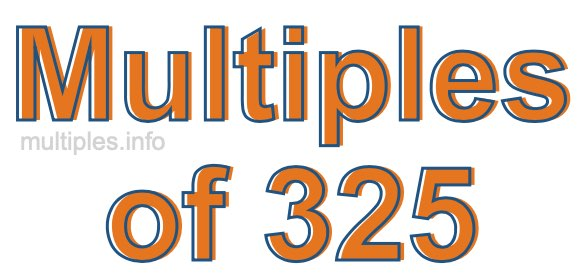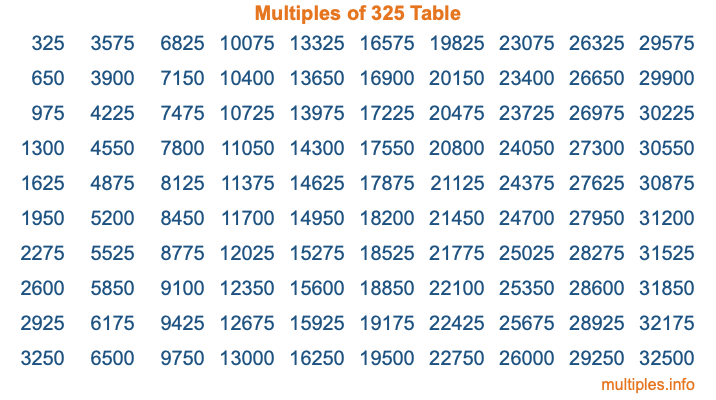Multiples of 325Welcome to the Multiples of 325 page. Here we will first teach you everything you will ever need to know about the multiples of 325, and then give you a study guide summary of everything we taught you to make sure you remember it all. Use this page to look up facts and learn information about the multiples of 325. This page will make you a multiples of three hundred twenty-five expert!

Definition of Multiples of 325
Multiples of 325 are all the numbers that when divided by 325 equal an integer. Each of the multiples of 325 are called a multiple. A multiple of 325 is created by multiplying 325 by an integer.

Therefore, to create a list of multiples of 325, you start with 1 multiplied by 325, then 2 multiplied by 325, then 3 multiplied by 325, and so on for as long as you want. Thus, the list of the first five multiples of 325 is 325, 650, 975, 1300, and 1625. To see a larger list of multiples of 325, see the printable image of Multiples of 325 further down on this page. We also have a category where you can choose any nth multiple of 325.

Multiples of 325 Checker
The Multiples of 325 Checker below checks to see if any number of your choice is a multiple of 325. In other words, it checks to see if there is any number (integer) that when multiplied by 325 will equal your number. To do that, we divide your number by 325. If the the quotient is an integer, then your number is a multiple of 325.

Is  a multiple of 325?

Least Common Multiple of 325 and ...
A Least Common Multiple (LCM) is the lowest multiple that two or more numbers have in common. This is also called the smallest common multiple or lowest common multiple and is useful to know when you are adding our subtracting fractions. Enter one or more numbers below (325 is already entered) to find the LCM.

Check out our LCM Calculator if you need more details about the Least Common Multiple or if you need the LCM for different numbers for adding and subtraction fractions.

nth Multiple of 325
As we stated above, 325 is the first multiple of 325, 650 is the second multiple of 325, 975 is the third multiple of 325, and so on. Enter a number below to find the nth multiple of 325.

th multiple of 325

Multiples of 325 vs Factors of 325
325 is a multiple of 325 and a factor of 325, but that is where the similarities end. All postive multiples of 325 are 325 or greater than 325. All positive factors of 325 are 325 or less than 325.

Below is the beginning list of multiples of 325 and the factors of 325 so you can compare:

Multiples of 325: 325, 650, 975, 1300, 1625, etc.

Factors of 325: 1, 5, 13, 25, 65, 325

As you can see, the multiples of 325 are all the numbers that you can divide by 325 to get a whole number. The factors of 325, on the other hand, are all the whole numbers that you can multiply by another whole number to get 325.

It's also interesting to note that if a number (x) is a factor of 325, then 325 will also be a multiple of that number (x).

Multiples of 325 vs Divisors of 325
The divisors of 325 are all the integers that 325 can be divided by evenly. Below is a list of the divisors of 325.

Divisors of 325: 1, 5, 13, 25, 65, 325

The interesting thing to note here is that if you take any multiple of 325 and divide it by a divisor of 325, you will see that the quotient is an integer.

Multiples of 325 Table
Below is an image of the first 100 multiples of 325 in a table. The table is in chronological order, column by column. The first column has the first ten multiples of 325, the second column has the next ten multiples of 325, and so on.The Multiples of 325 Table is also referred to as the 325 Times Table or Times Table of 325. You are welcome to print out our table for your studies.

Negative Multiples of 325
Although not often discussed or needed in math, it is worth mentioning that you can make a list of negative multiples of 325 by multiplying 325 by -1, then by -2, then by -3, and so on, to get the following list of negative multiples of 325:

-325, -650, -975, -1300, -1625, etc.

Multiples of 325 Summary
Below is a summary of important Multiples of 325 facts that we have discussed on this page. To retain the knowledge on this page, we recommend that you read through the summary and explain to yourself or a study partner why they hold true.

There are an infinite number of multiples of 325.

A multiple of 325 divided by 325 will equal a whole number.

325 divided by a factor of 325 equals a divisor of 325.

The nth multiple of 325 is n times 325.

The largest factor of 325 is equal to the first positive multiple of 325.

325 is a multiple of every factor of 325.

325 is a multiple of 325.

A multiple of 325 divided by a divisor of 325 equals an integer.

325 divided by a divisor of 325 equals a factor of 325.

Any integer times 325 will equal a multiple of 325.

Multiples of a Number
Here you can get the multiples of another number, all with the same attention to detail as we did for multiples of 325 on this page.

Multiples of
Multiples of 326
Did you find our page about multiples of three hundred twenty-five educational? Do you want more knowledge? Check out the multiples of the next number on our list!